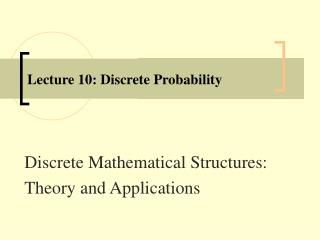DownloadDownload PresentationLecture 10: Discrete Probability

# Lecture 10: Discrete Probability

Télécharger la présentation## Lecture 10: Discrete Probability

- - - - - - - - - - - - - - - - - - - - - - - - - - - E N D - - - - - - - - - - - - - - - - - - - - - - - - - - -
##### Presentation Transcript

1. Lecture 10: Discrete Probability Discrete Mathematical Structures: Theory and Applications

2. Learning Objectives • Learn the basic counting principles—multiplication and addition • Explore the pigeonhole principle • Learn about permutations • Learn about combinations Discrete Mathematical Structures: Theory and Applications

3. Learning Objectives • Explore generalized permutations and combinations • Learn about binomial coefficients and explore the algorithm to compute them • Discover the algorithms to generate permutations and combinations • Become familiar with discrete probability Discrete Mathematical Structures: Theory and Applications

4. Discrete Probability • Definition 7.8.1 • A probabilistic experiment, or random experiment, or simply an experiment, is the process by which an observation is made. • In probability theory, any action or process that leads to an observation is referred to as an experiment. • Examples include: • Tossing a pair of fair coins. • Throwing a balanced die. • Counting cars that drive past a toll booth. Discrete Mathematical Structures: Theory and Applications

5. Discrete Probability • Definition 7.8.3 • The sample space associated with a probabilistic experiment is the set consisting of all possible outcomes of the experiment and is denoted by S. • The elements of the sample space are referred to as sample points. • A discrete sample space is one that contains either a finite or a countable number of distinct sample points. Discrete Mathematical Structures: Theory and Applications

6. Discrete Probability • Definition 7.8.6 • An event in a discrete sample space S is a collection of sample points, i.e., any subset of S. In other words, an event is a set consisting of possible outcomes of the experiment. • Definition 7.8.7 • A simple event is an event that cannot be decomposed. Each simple event corresponds to one and only one sample point. Any event that can be decomposed into more than one simple event is called a compound event. Discrete Mathematical Structures: Theory and Applications

7. Discrete Probability • Definition 7.8.8 • Let A be an event connected with a probabilistic experiment E and let S be the sample space of E. The event B of nonoccurrence of A is called the complementary event of A. This means that the subset B is the complement A’of A in S. • In an experiment, two or more events are said to be equally likely if, after taking into consideration all relevant evidences, none can be expected in reference to another. Discrete Mathematical Structures: Theory and Applications

8. Discrete Probability Discrete Mathematical Structures: Theory and Applications

9. Discrete Probability • Axiomatic Approach • Analyzing the concept of equally likely probability, we see that three conditions must hold. • The probability of occurrence of any event must be greater than or equal to 0. • The probability of the whole sample space must be 1. • If two events are mutually exclusive, the probability of their union is the sum of their respective probabilities. • These three fundamental concepts form the basis of the definition of probability. Discrete Mathematical Structures: Theory and Applications

10. Discrete Probability Discrete Mathematical Structures: Theory and Applications

11. Discrete Probability Discrete Mathematical Structures: Theory and Applications

12. Discrete Probability Discrete Mathematical Structures: Theory and Applications

13. Discrete Probability • Conditional Probability • Consider the throw of two distinct balanced dice. To find the probability of getting a sum of 7, when it is given that the digit in the first die is greater than that in the second. • In the probabilistic experiment of throwing two dice the sample space S consists of 6 * 6 = 36 outcomes. • Assume that each of these outcomes is equally likely. Let A be the event: The sum of the digits of the two dice is 7, and let B be the event: The digit in the first die is greater than the second. Discrete Mathematical Structures: Theory and Applications

14. Discrete Probability • Conditional Probability • A : {(6, 1), (5 , 2), (4, 3), (3, 4), (2, 5), (1, 6)} • B : {(6, 1), (6, 2), (6, 3), (6, 4), (6, 5), (5 , 1), (5 , 2), (5 , 3),(5 , 4), (4, 1), (4, 2), (4, 3), (3, 1), (3, 2), (2, 1)}. • Let C be the event: The sum of the digits in the two dice is 7 but the digit in the first die is greater than the second. Then C : {(6, 1), (5 , 2), (4, 3)} = A ∩ B. Discrete Mathematical Structures: Theory and Applications